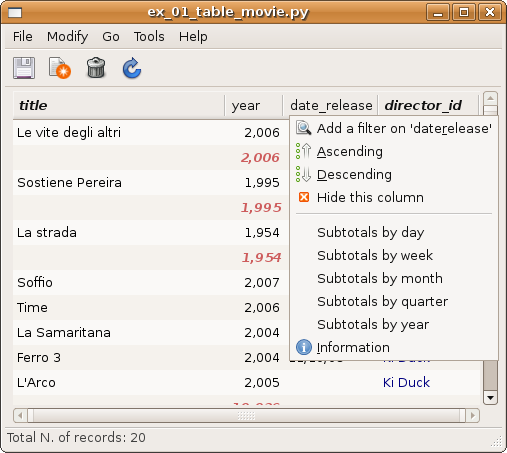### Questions?

Subscribe to out mailing list Google group:

# Totals¶

Numeric columns of a Table have the option to show totals and subtotals. Subtotals are determined by brake fields/functions. This may be done interactively from the column menu or from code. The following example is very stupid but... you get the feeling!:

```t = SqlTable('movie', dbproxy=db, order_by='director_id')
```## Personalization and colors¶

Totals are generated by a class sqlkit.widgets.table.totals.Totals that can be inherited and modified to set different total behavior.

The colors are defined in a class in the same module TotalObj whose method set_value_and_colors can be used to personalize colors and markup of the cell.

## Dates¶

Since date breaks are probably very common a function makes it easy to brake on dates.

## Totals... with no totals!¶

If you only need subtotals and not totals you can prevent totals using option hide_total when declaring the column to sum.

## Signals¶

computed:
this signal is emitted when the total is computed. The signature

of the callback function is:

computed_cb(total):
Parameter: sqlwidget – the total instance that emitted the signal

### API¶

class sqlkit.widgets.table.totals.Totals(table, treeview=None)

An object whose ‘compute’ method adds TotalObjets to show partial totals/grand totals to a table. A TotalObject is inserted each time total_by changes. If total_by is a callable, it’s evaluated to detect if a partial total must be inserted

totals
A dict whose keys are the field names for which a total/subtotal is to be computed. The values are the totals. It’s filled by sum() that can be customized.
subtotals
A dict whose keys are the field names for which a total/subtotal is to be computed The values are the subtotals. It’s filled by sum() that can be customized.

Add a total object each time subtotal changes

Parameters: field_names – a list of field_names for which we want a total quiet – boolean. If True silently fail if the field is not a number hide_total – boolean. If True gran total will not be shown (only subtotals)

If no func is provided, a break_func will be setup to insert a subtotal each time field_value changes.

Othewise func will be set as break_function. Break function must have this signature:

`def break_func(obj, field_name, path)`

see func_date_* is this module for examples

Set date break period can be: day, week, month, quarter, year
compute()
sum(obj, model, path, iter)

sum values and store result in totals and subtotals

Parameters: obj – the obj that should be added. It’s an instance of table.mapper.class_ model – the model of the treeview path – the path at which the obj is iter – the iter at which the obj is

To customize the behaviour of total you can just customize this method, suppose you want to make it sum flagged objects in table t:

```class BoolTotals(totals.Totals):

def sum(self, obj, model, path, iter):
if obj.flag:
for field_name in self.totals.keys():
self.totals[field_name]    += getattr(obj, field_name, 0) or 0
self.subtotals[field_name] += getattr(obj, field_name, 0) or 0

t. = SqlTable(...)
t.totals = BoolTotal(t)```
class sqlkit.widgets.table.totals.TotalObj(fields, sub=True)

A simple class that represents the object holding the totals and a way to represent it

personalization

You can change the look of totals inheriting from TotalObj and placing the new class as class attribute of Totals

set_value_and_colors(cell, value, field_name)
set the color cell and possibly background